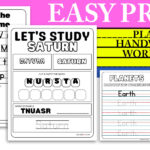# 25+ Fraction Division Worksheet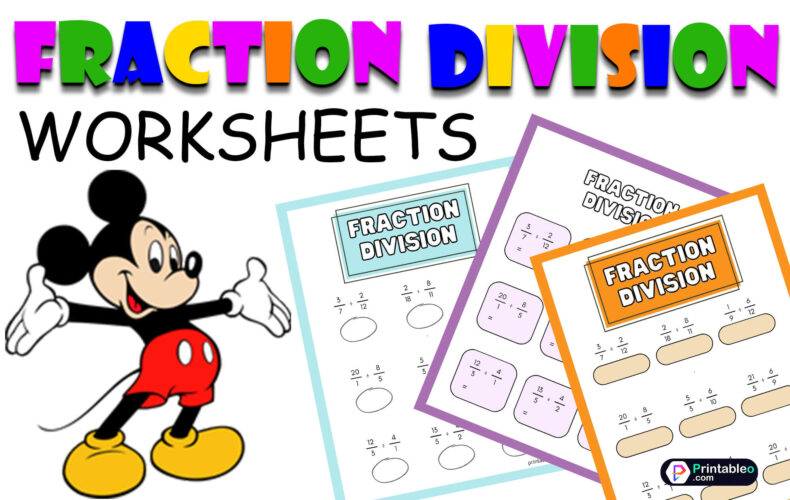Division means to share equally; after learning about the division of whole numbers, our next topic is always to know about fraction divisions with a few easy steps to do it. A fraction has two parts, namely a numerator, or the digit on the top, and a denominator, or the bottom digit.

Practicing on worksheets can help in increasing a student’s knowledge on a topic, so these printable Fraction Division Worksheets enlisted below can be made into exercise notes or used as tests by printing them with the easy download options, and you can customize them if needed.

Dividing fractions is quite similar to the multiplication of fractions. We have to find out the reciprocal of a fraction, which is calculated by inverting it or exchanging the position of the digits by placing the numerator at the bottom and the denominator above it.

Once we have got the reciprocated fraction, we can simply follow the steps of Fraction multiplication, which is to multiply all the numerators with each other, and do the same with all the denominators and finally place the end results in their respective places. Some fractions can be simplified after multiplication.

This compilation of 25+ worksheets on Fraction Division has different combinations of numbers, helping children to practice with different possibilities and gain a clear knowledge of the topic. They have child-friendly, simple designs to make studying easier and more exciting.

## Fraction Division Worksheets: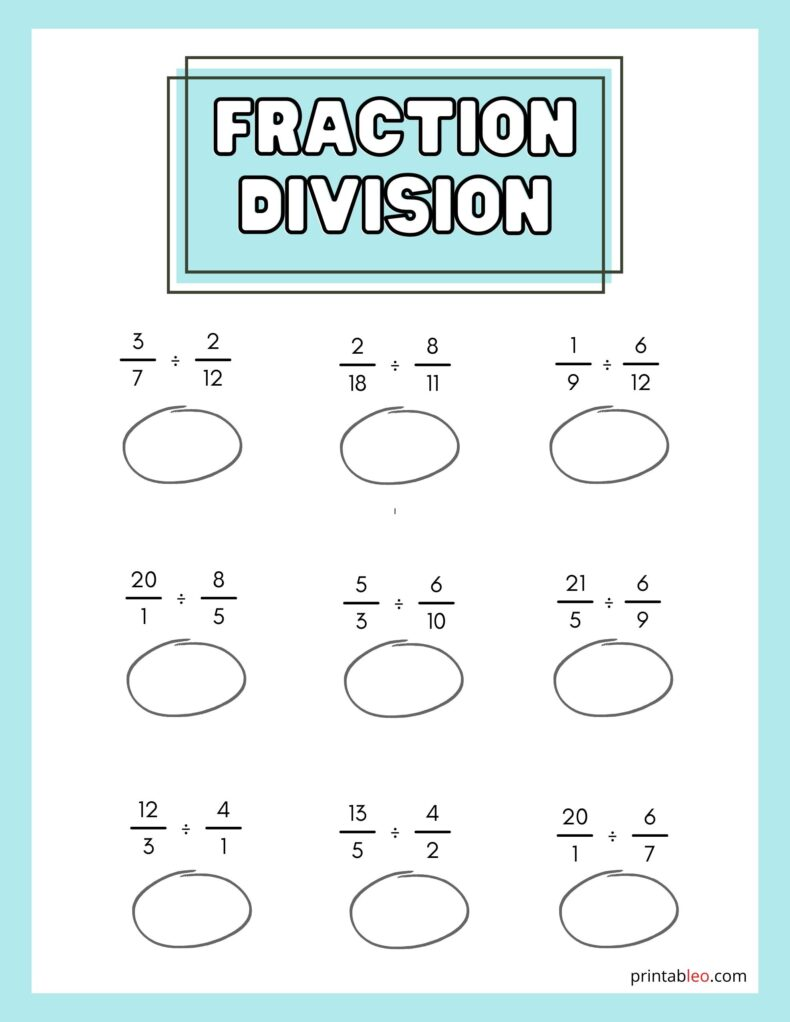#### 2. Worksheets On Division Of Fractions:

This simple, light-toned worksheet on Fraction Division has simple division exercises suitable for a new learner, who can calculate the results and cleanly write them in the provided hand-drawn circle under each question.

For preschoolers and primary school pupils who are learning fractions or students who need practice on fraction divisions, this collection of Division And Fraction Worksheets might help them practice the steps regularly, gaining more confidence by understanding the topic clearly.

The above-listed Worksheets on Division Of Fractions printable has a simple outlook with 12 unique questions, whose results can be placed beside the ‘equal to’ sign adjacent to every numerical sum. It has a varied set of fractions to practice more efficiently.

This worksheet has included a space for mentioning name and date, making it perfect for places with more than one student, like schools, tutorial centers, and daycares, and even for homes that have preschoolers or primary school students living together, like siblings, twins or foster cares.

## How to Do Fraction Division:(Method)

 In order to divide two fractions, you first need to express or figure the fractions with a common denominator. For example, if you want to divide 1/2 by 1/3, the first thing you would need is to express the fractions with a common denominator and that is 6: 1/2 = 3/6 1/3 = 2/6 Now you can divide the numerators (the part of a fraction above the line) and denominators (the part of a fraction down the line) separately: (3/6) / (2/6) = (3/2) / (2/1) = 3/2 So the result of 1/2 divided by 1/3 is 3/2. Here’s another example: If you are trying to divide 3/4 by 2/5, first express the fractions with a common denominator which we will get by multiplying the denominators  i.e. 20: 3/4 = 15/20 2/5 = 8/20 Now divide the numerators and denominators separately: (15/20) / (8/20) = (15/8) / (8/8) = 15/8 So the end result of 3/4 divided by 2/5 is 15/8.

#### 3. Worksheet On Division Of Fractions: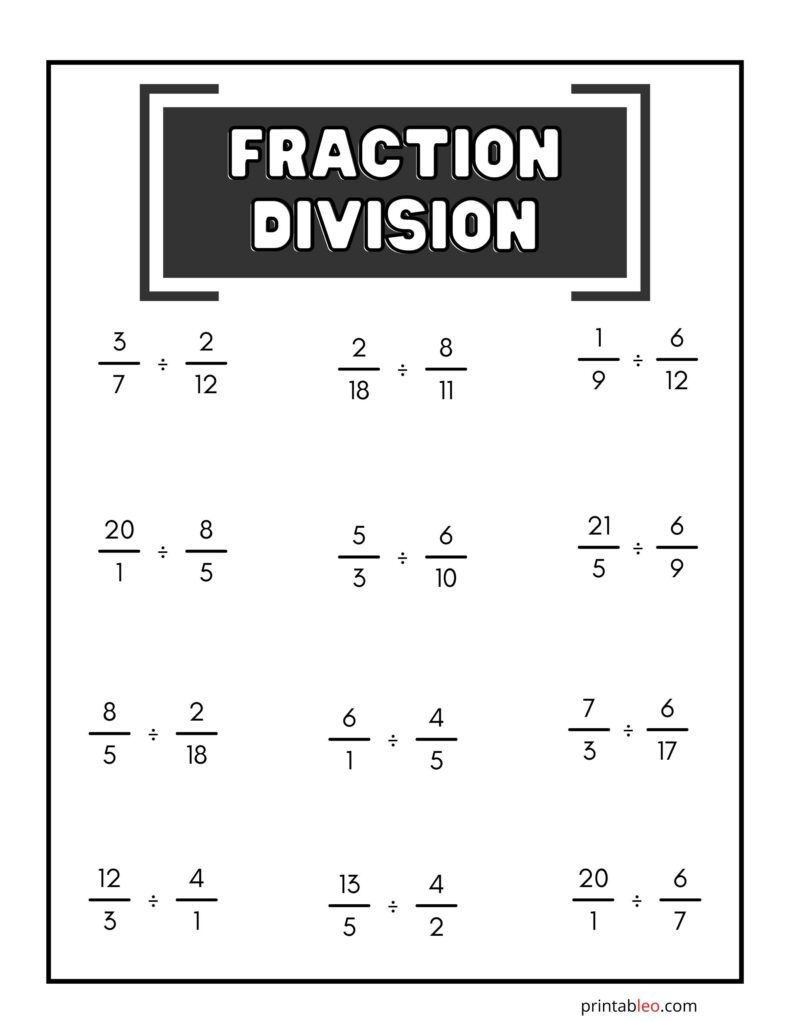#### 4. Relating Fractions To Division Worksheets: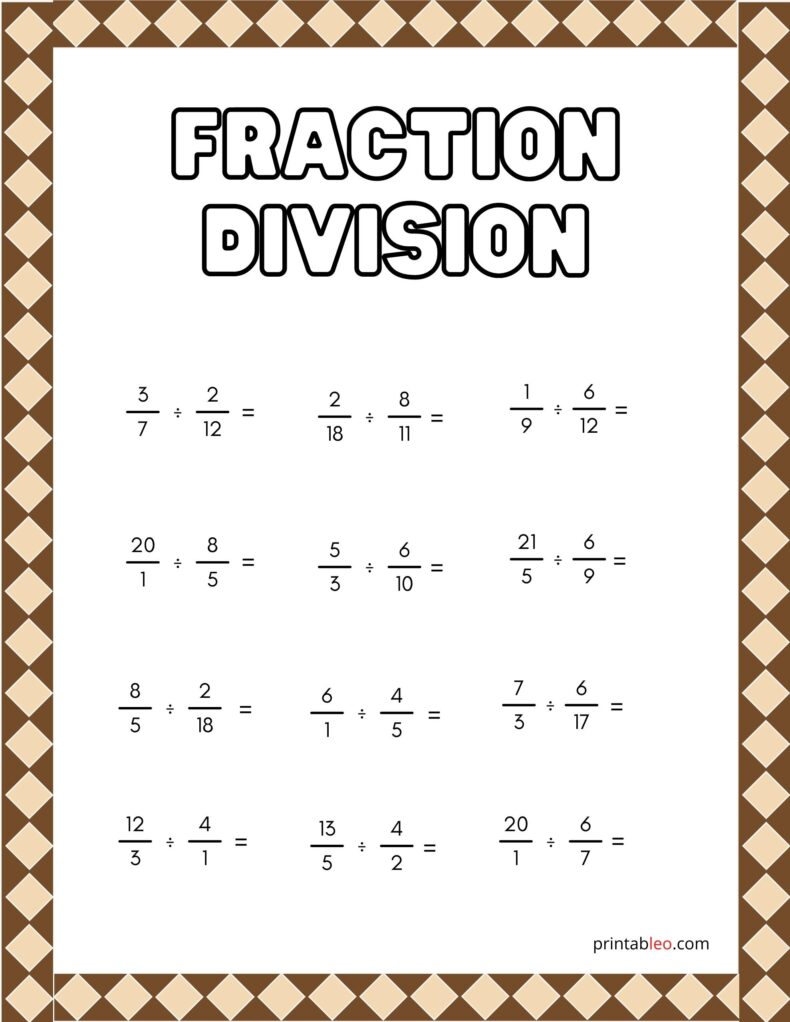#### 5. Printable Fractions To Division Worksheets: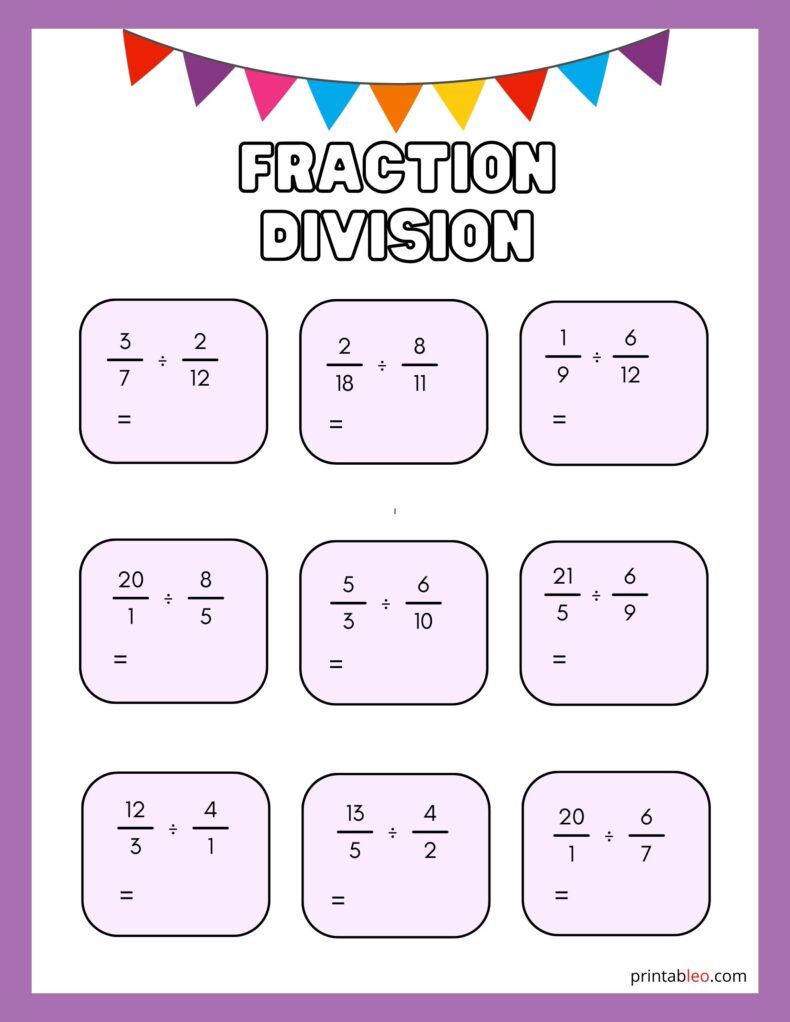This Printable Fractions To Division Worksheets has a colorful and vibrant look which can help to attract the children, making mathematics a bit more enjoyable. Each problem is placed in a box, where there is space to input the end results. It also has a party-flyer animation, making math more playful.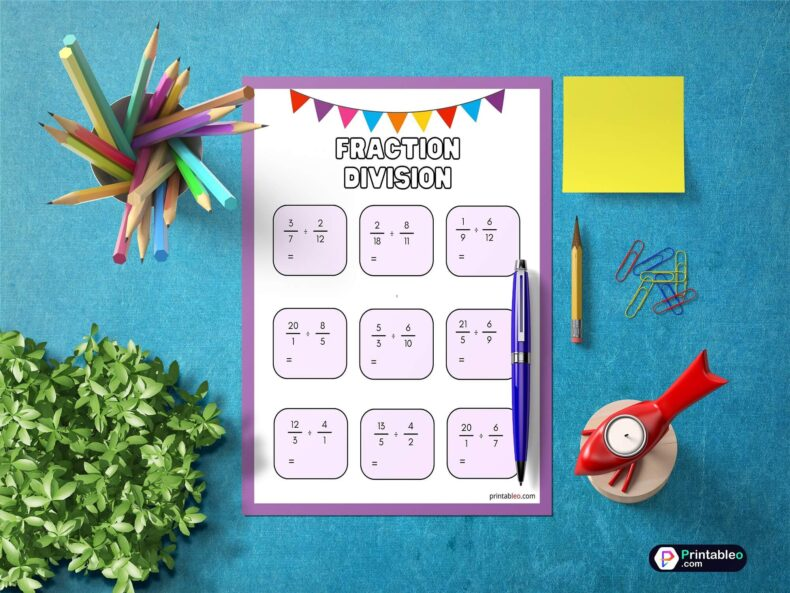#### 6. Modeling Fraction Division Worksheet: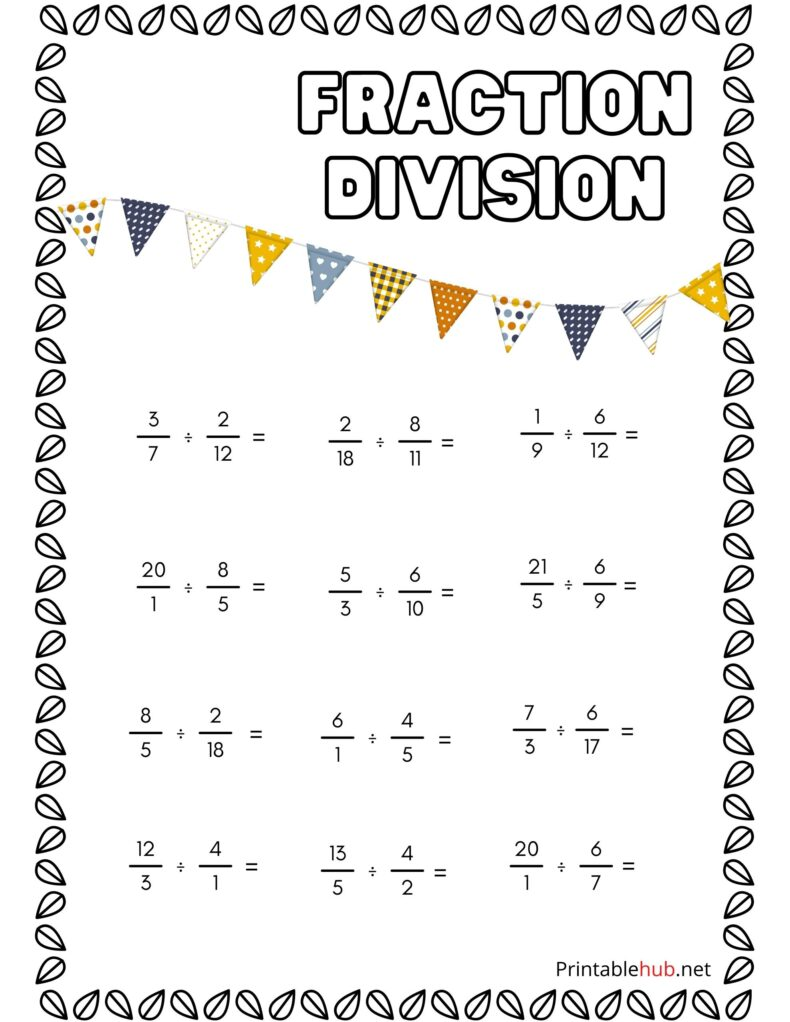#### 7. Fractions Division Worksheets: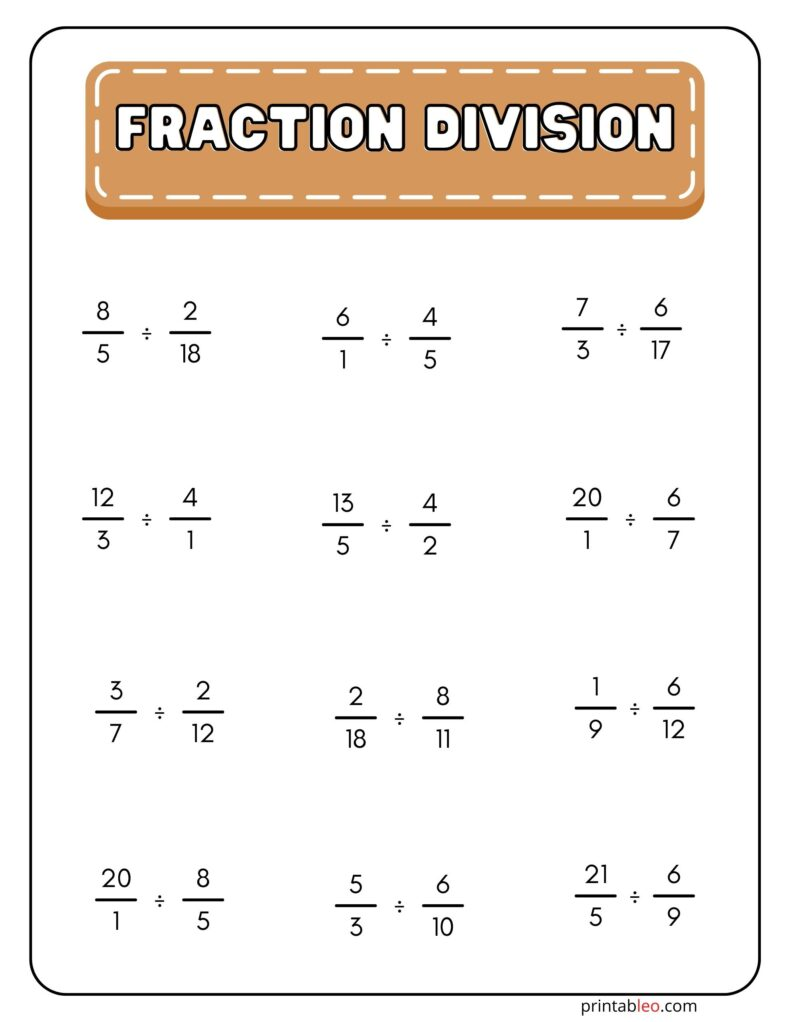#### 8. Fractions Division Worksheet: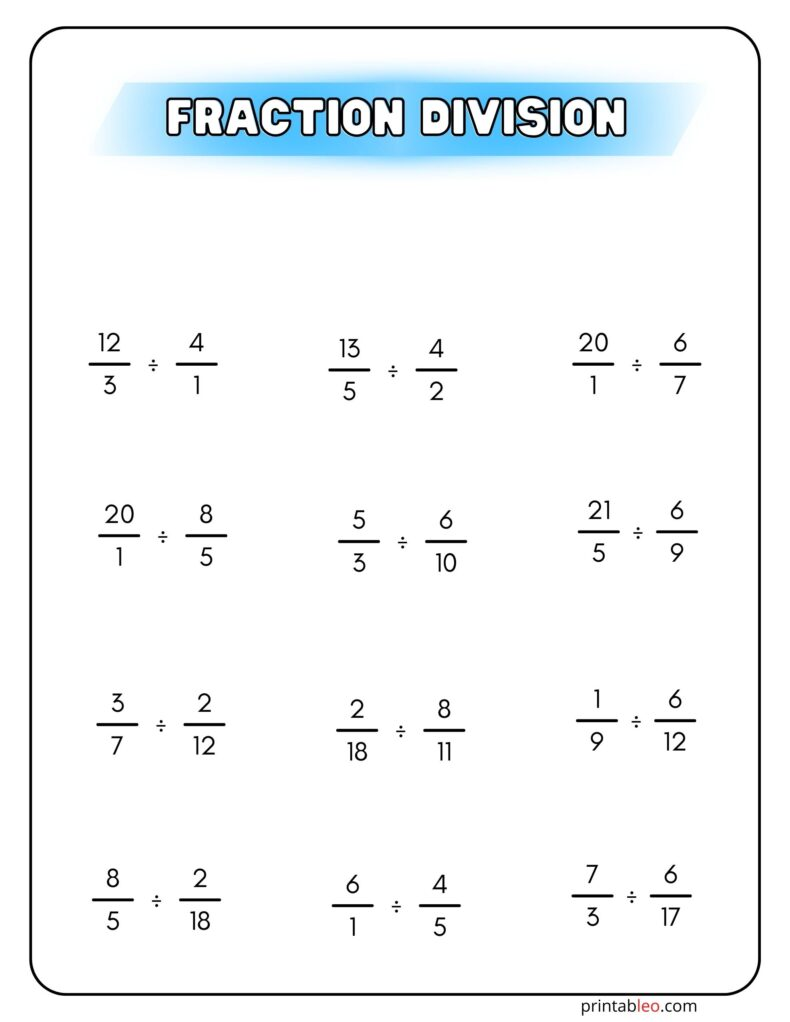#### 9. Fractions As Division Worksheets: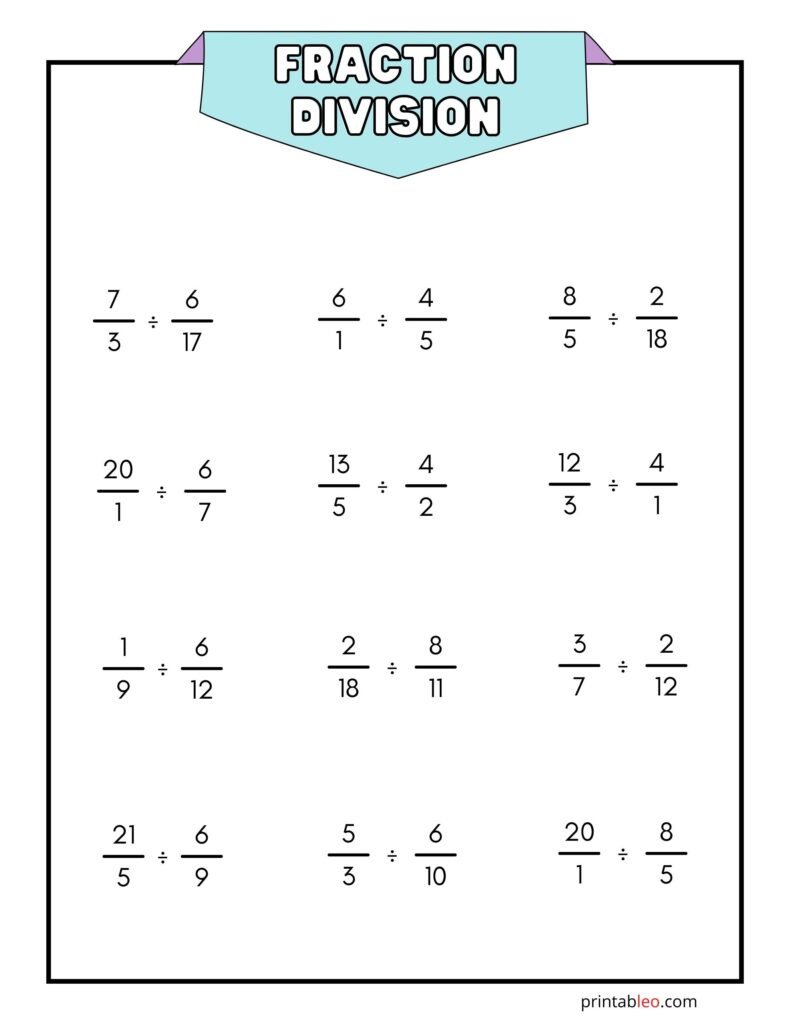#### 10. Fractions As Division Worksheet: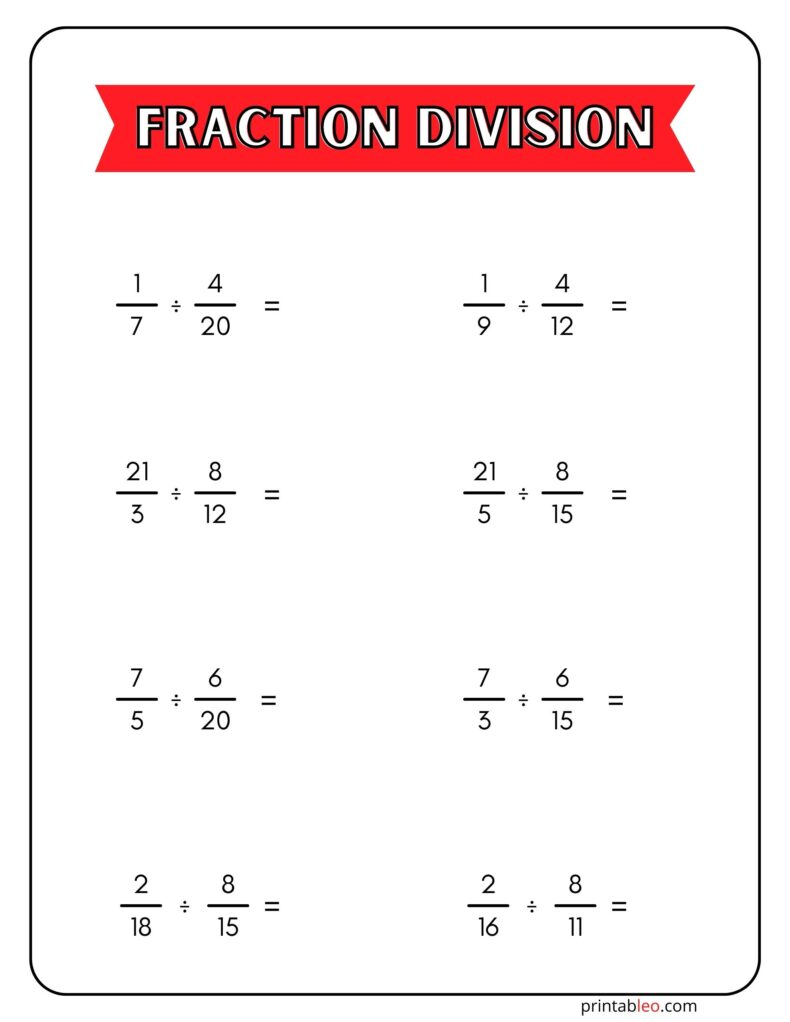#### 11. Fractions As Division Problems Worksheets: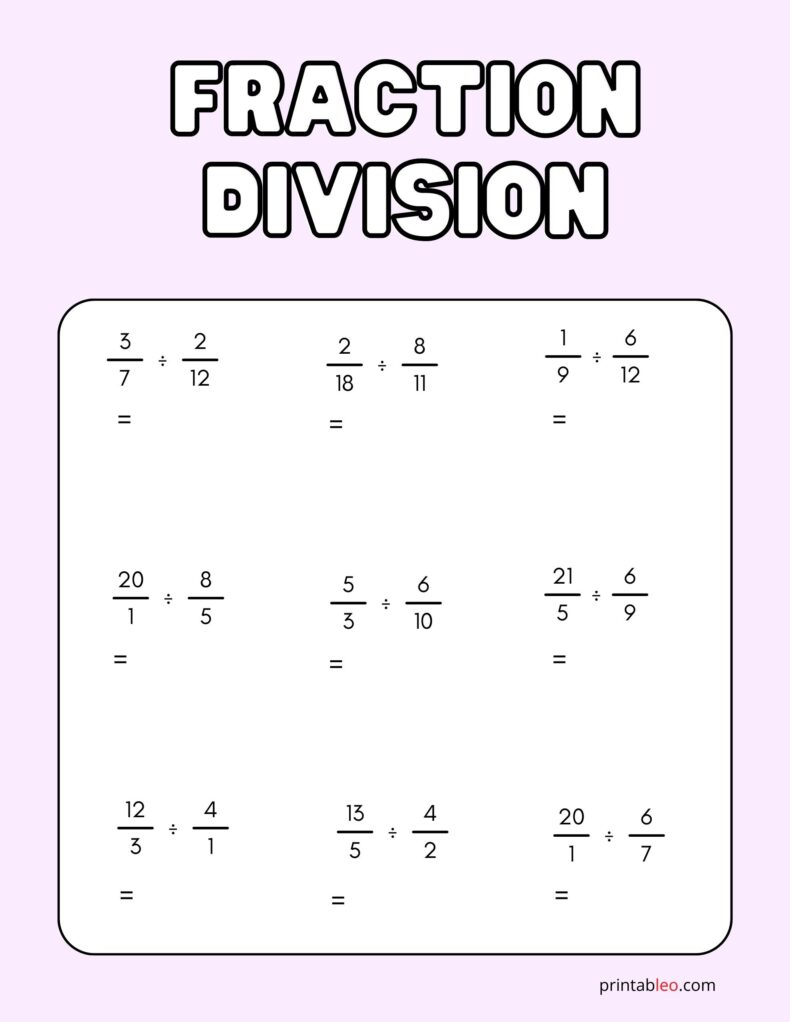#### 12. Fractions And Division Worksheets: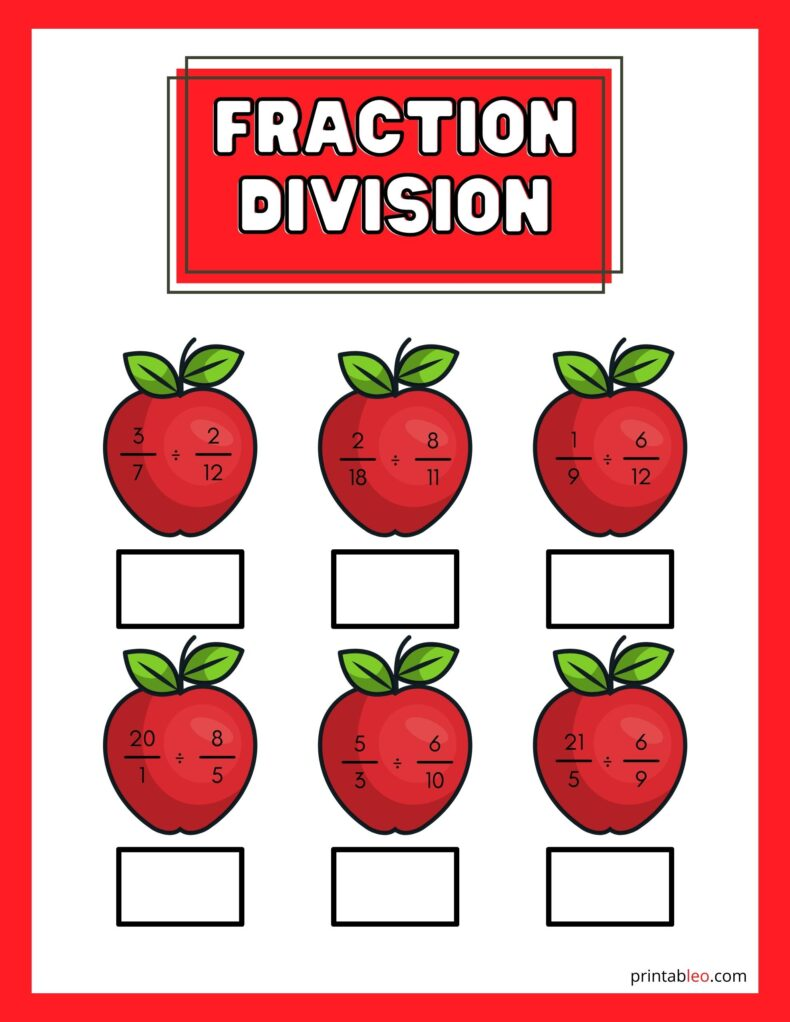The Fractions And Division Worksheet has an adorable representation, perfect for toddlers and children, as it inflicts a fun side of math exercises with the animated apples and vibrant colors. Each question has a separate box underneath, making it clear to the students to place the answers in it.

Adding visual appeal to children’s worksheets can evidently increase productivity while maintaining a happier mood, as they find excitement in learning through fun activities.

#### 13. Fraction Division Worksheets: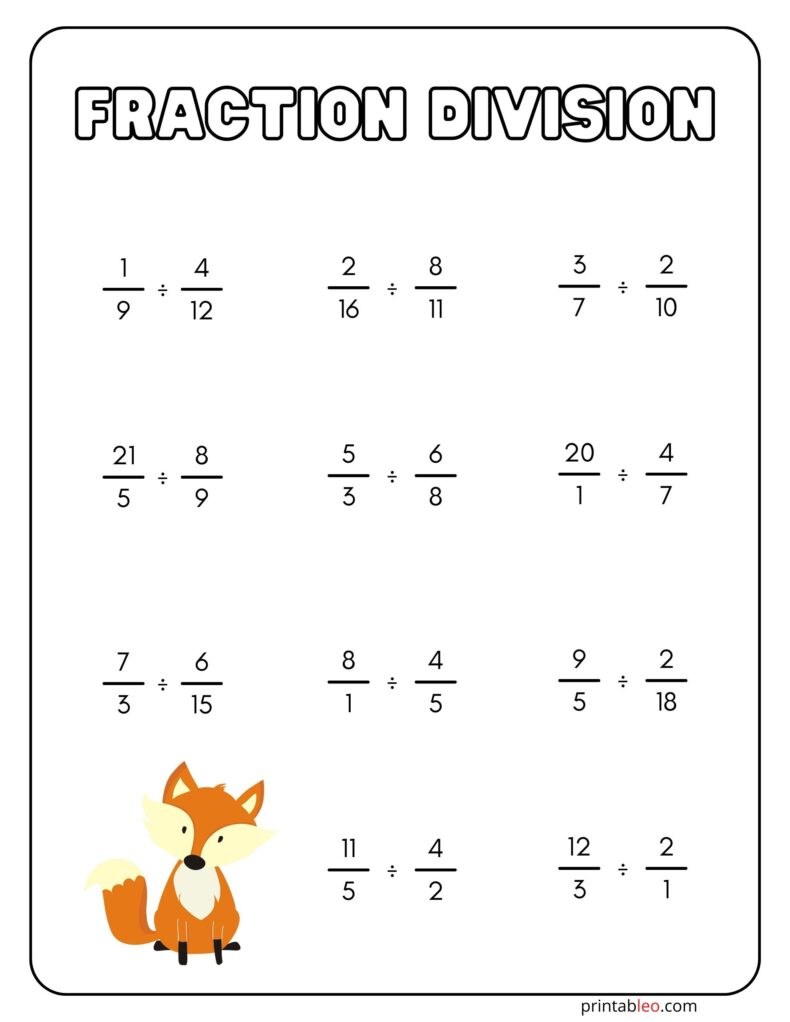A simple worksheet makes the questions clear to the children, and adding an animated animal to it increases their excitement while solving the problems. Children find joy in the slightest things, so this worksheet is perfect for primary school pupils and preschoolers.

#### 14. Fraction Division Worksheets Free: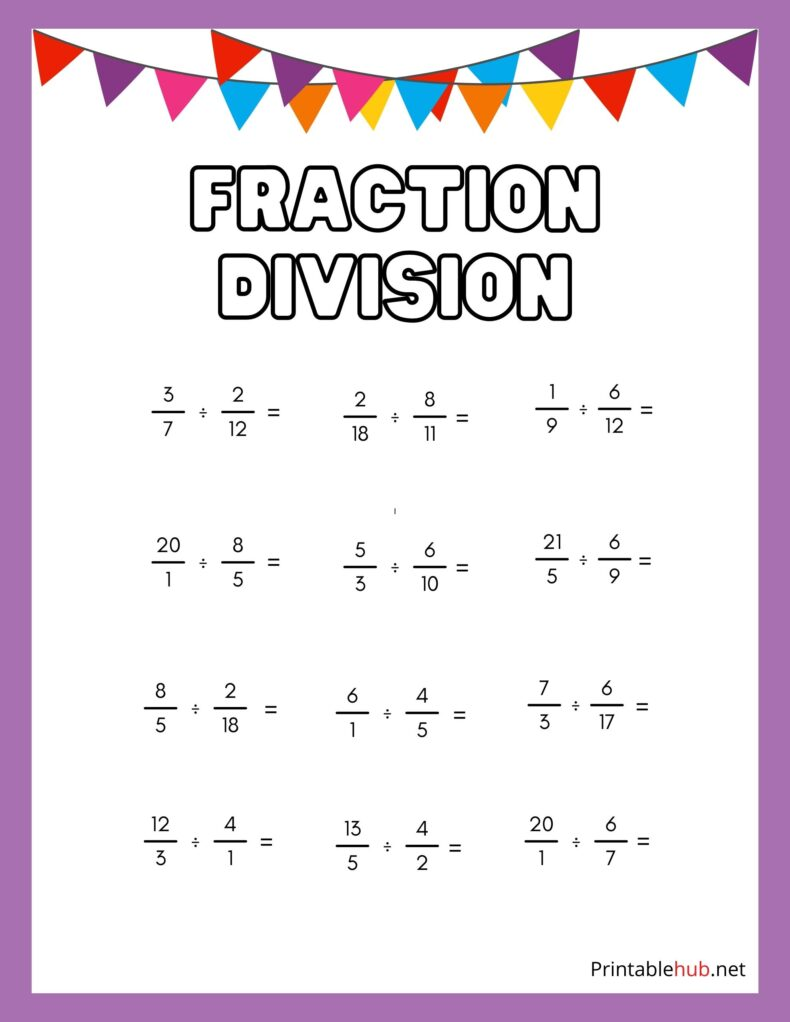#### 15. Fraction Division Worksheets 6th Grade: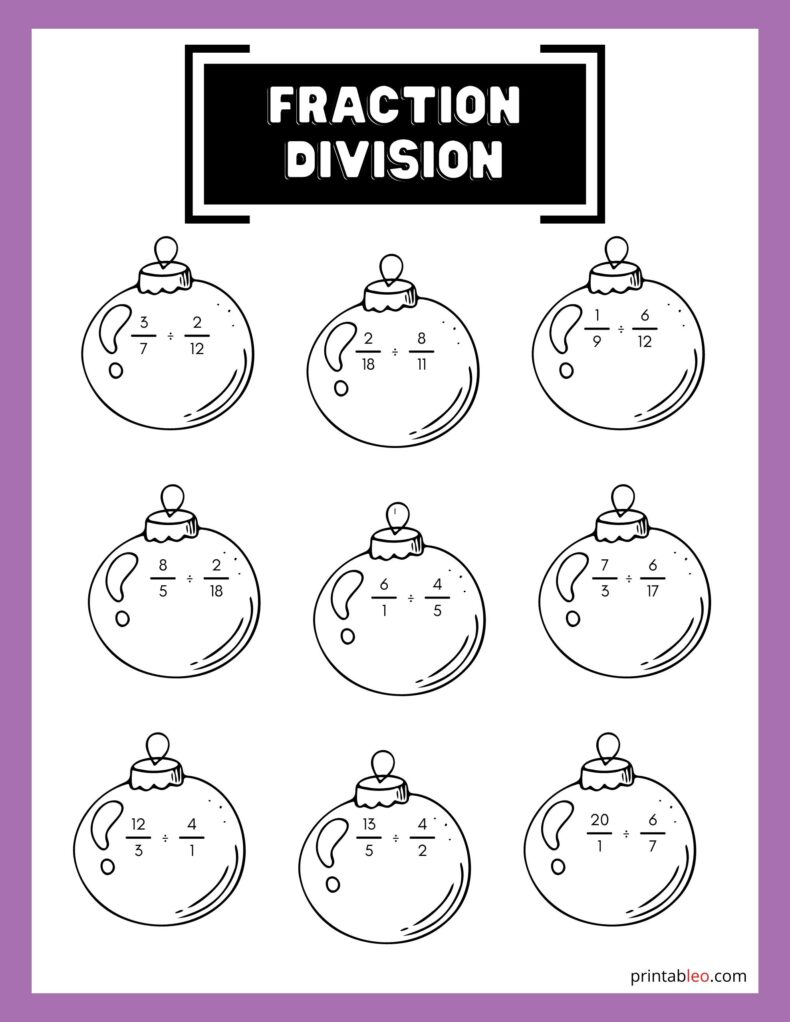#### 16. Fraction Division Worksheets 5th Grade: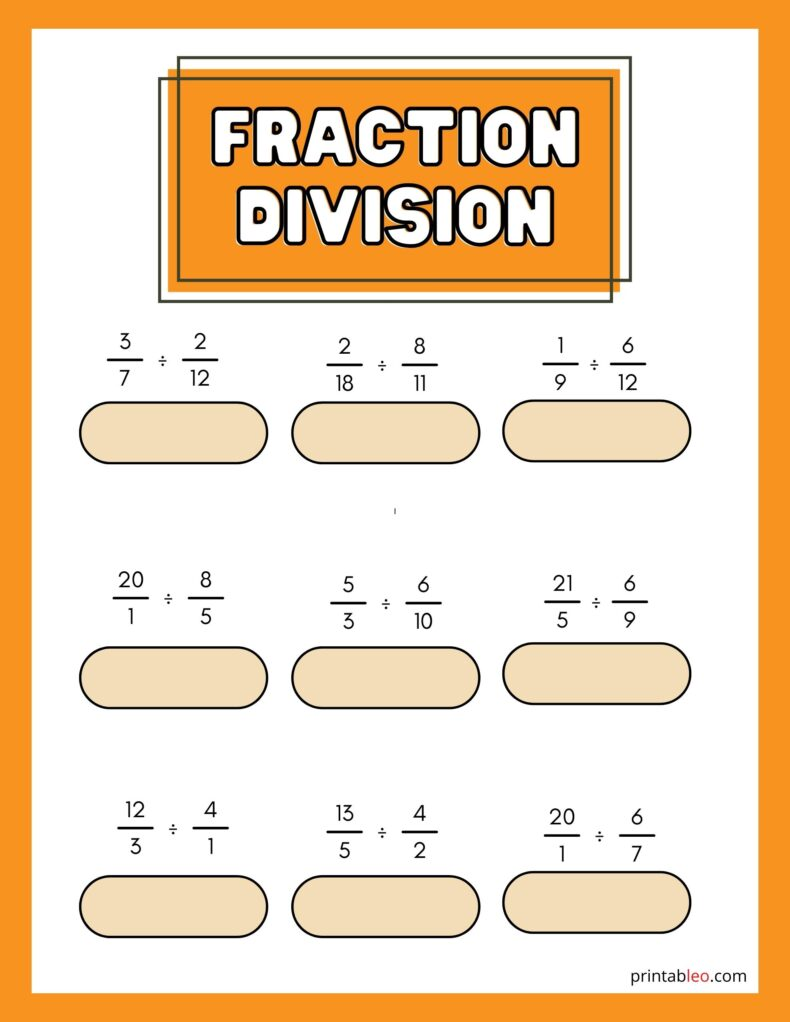## 17. Fraction Division Worksheet PDF: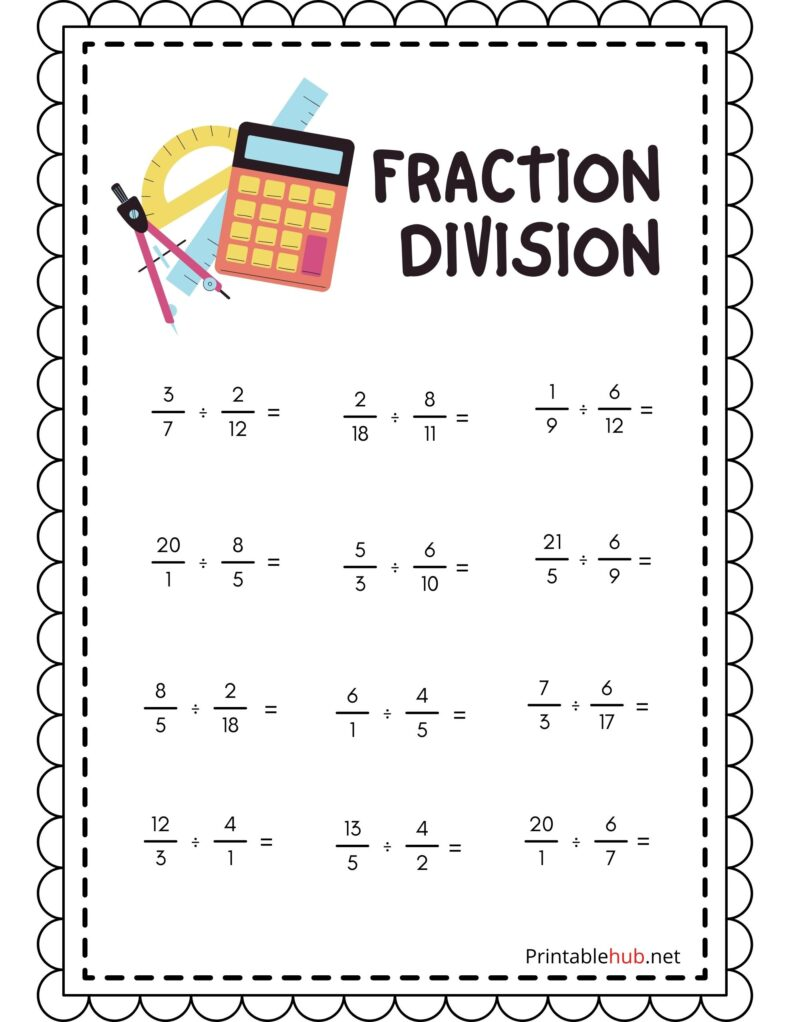## 18. Division With Fractions Worksheet: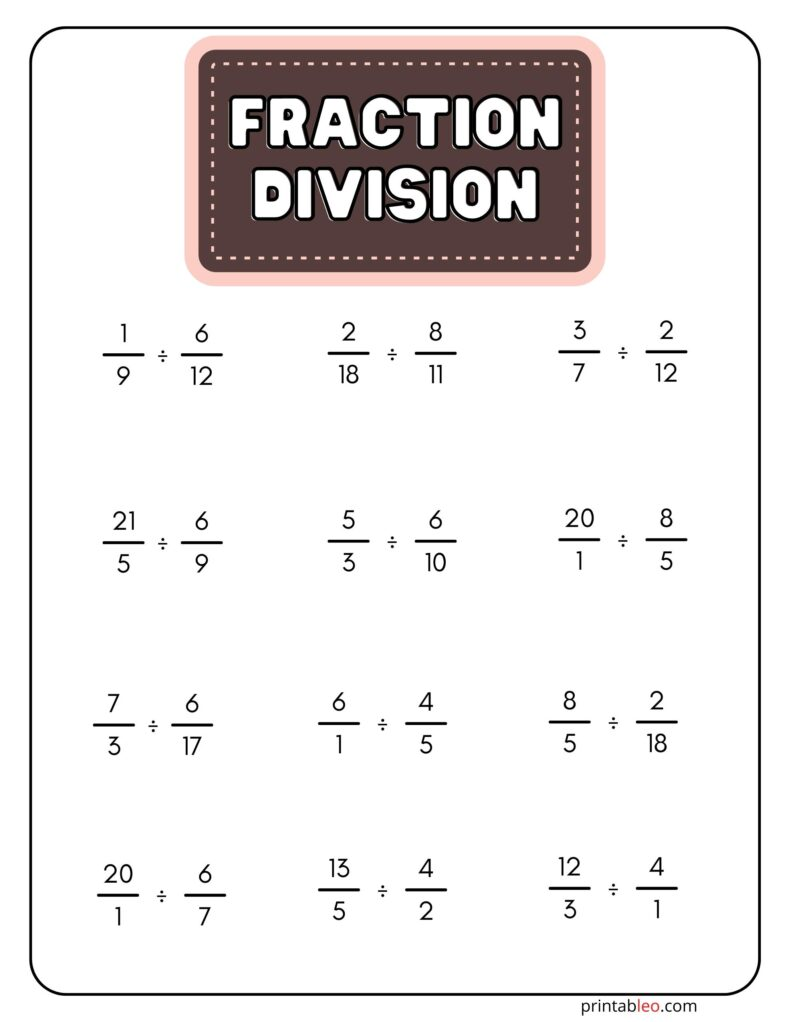## 19. Division With Fraction Remainders Worksheets: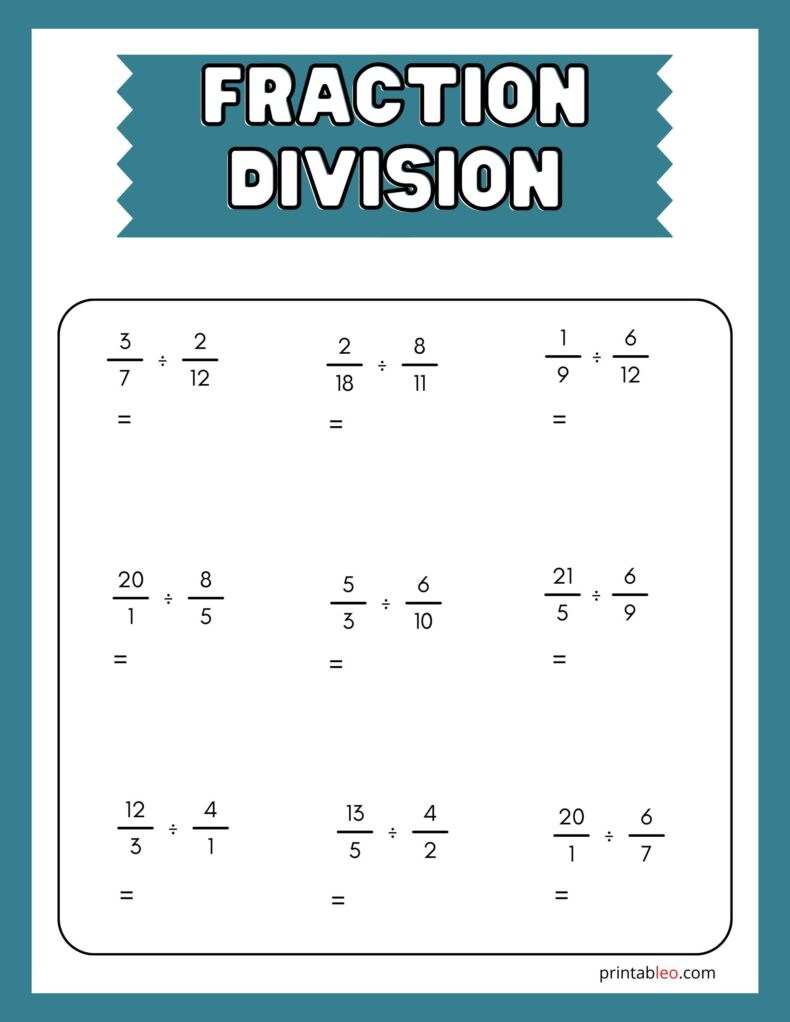## 20. Division Of Fractions Worksheets: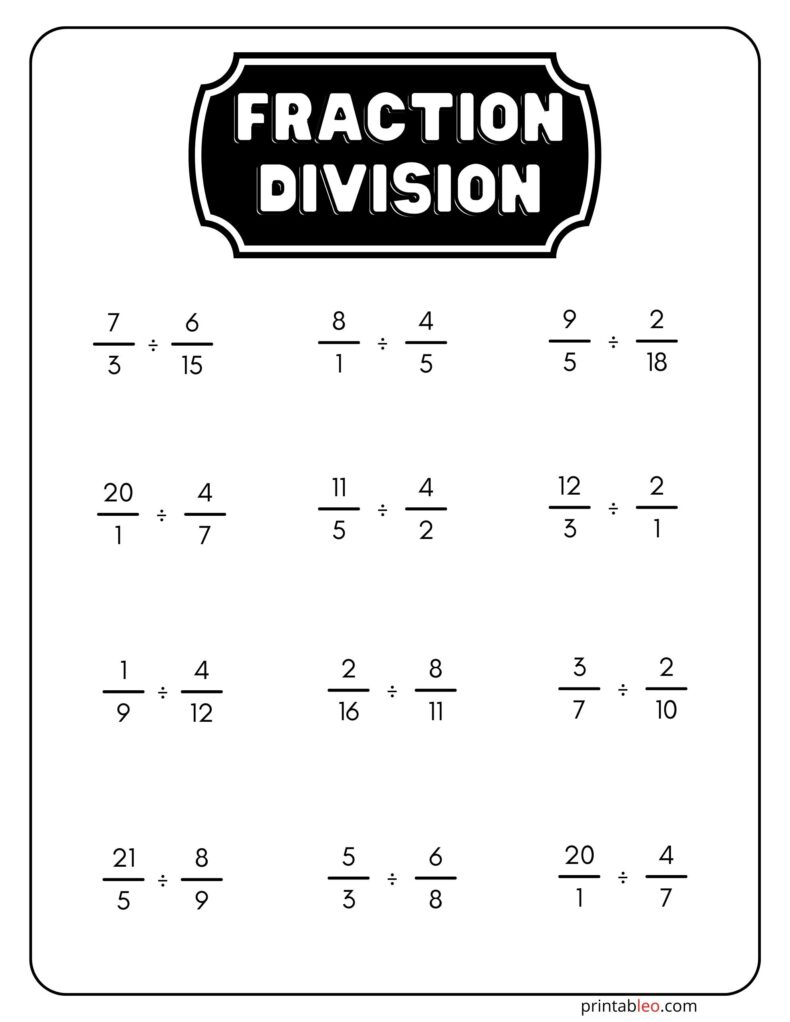## 21. Division Of Fractions Worksheets Grade 5: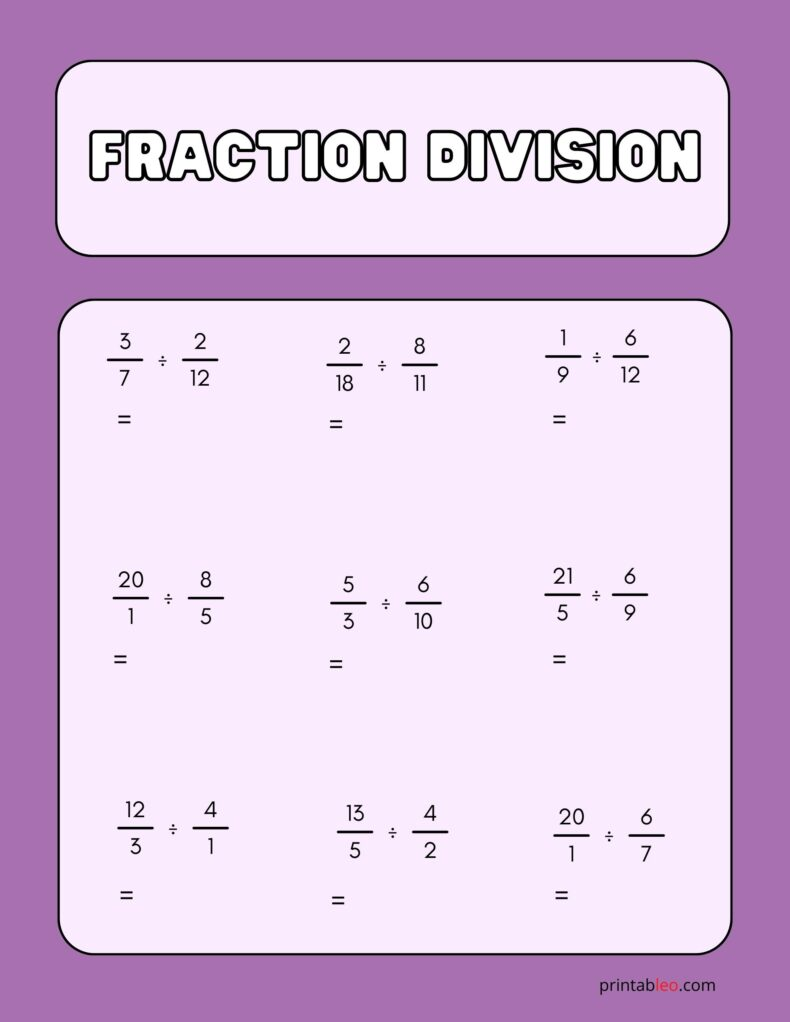Many toddlers and children have little attention spans, so they tend to get bored easily. Math problems can get boring and lead to chaos if the child does not enjoy the learning procedure. Using a colorful, bright worksheet with a simple design might be the perfect setup for such situations.

This Division Of Fractions Worksheets Grade 5 is perfect for maintaining visual appeal for students and can be mass printed as daily exercises, test papers, or practice sheets in schools, nurseries, or for domestic use.

## 22. Division Of Fractions Worksheets 6th Grade: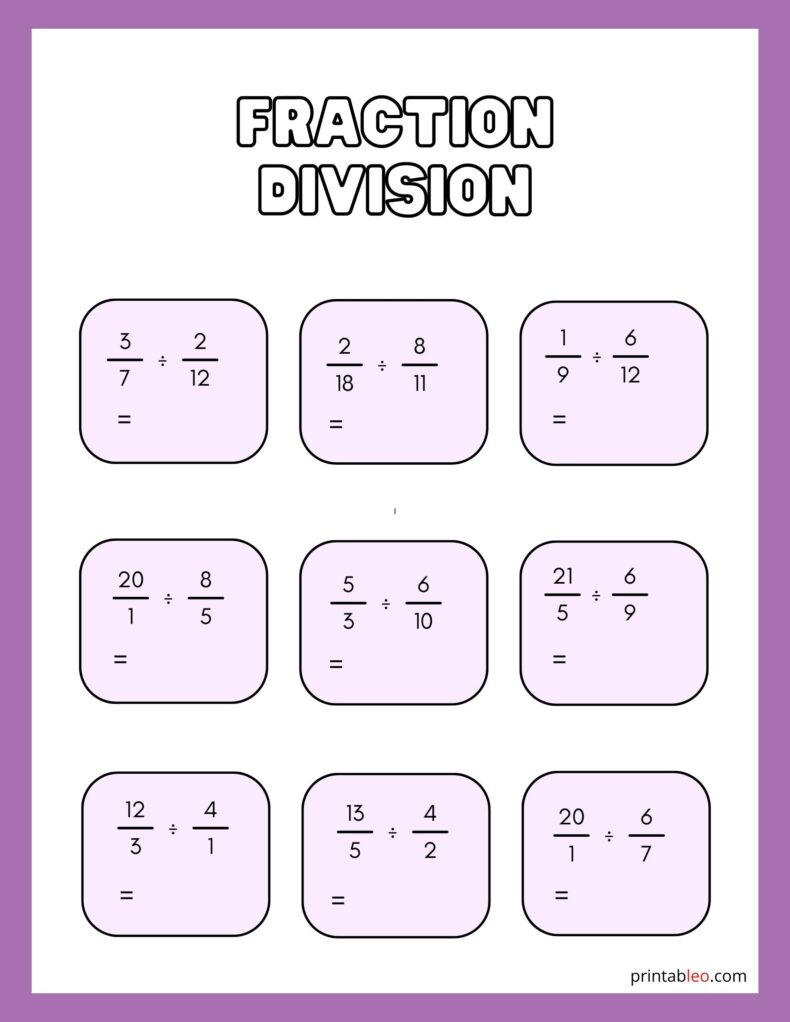## 23. Division Of Fractions Worksheet: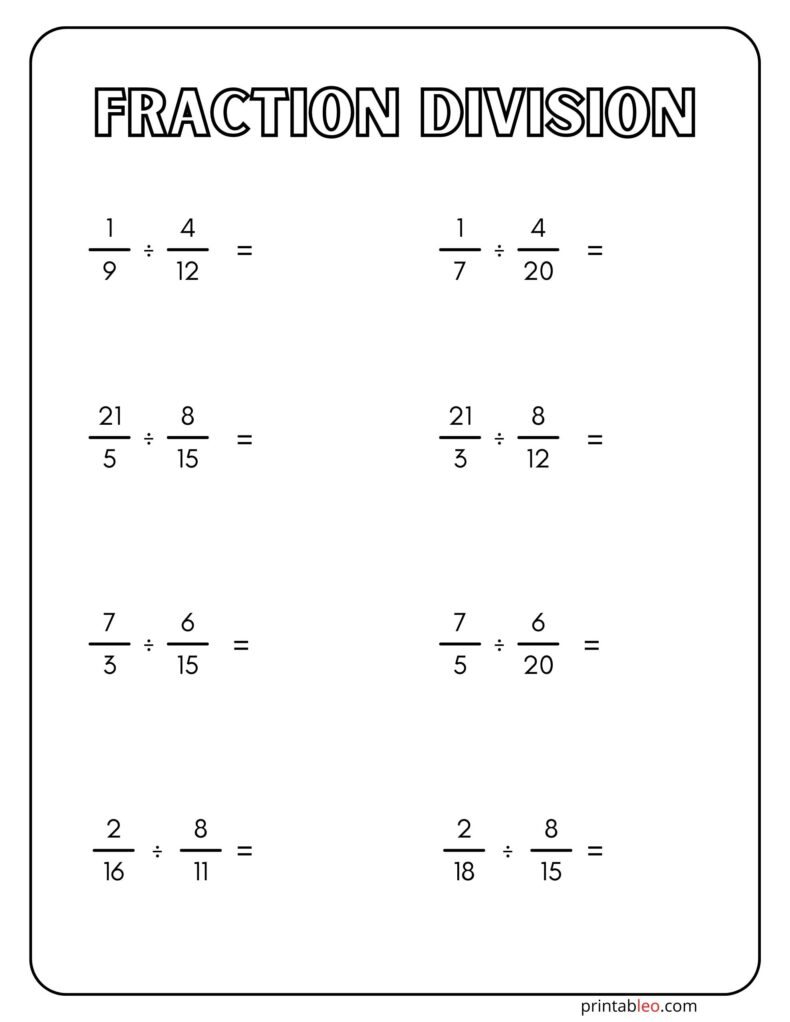## 24. Division Fractions Worksheets: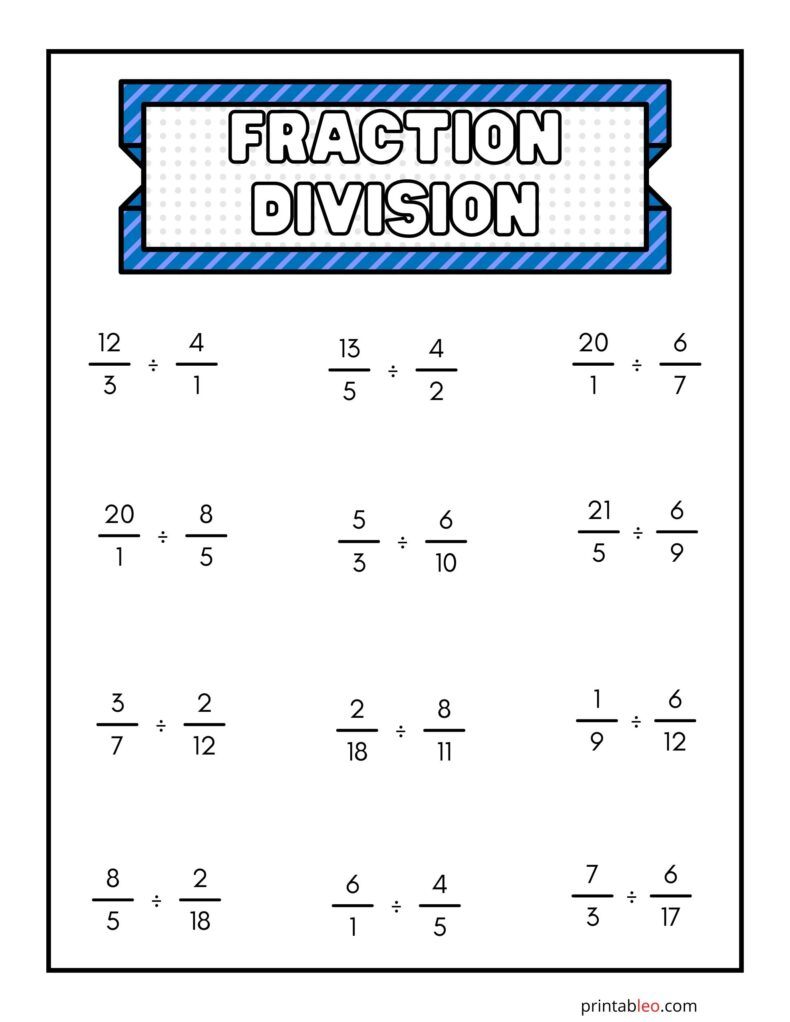## 25. Division As Fractions Worksheets: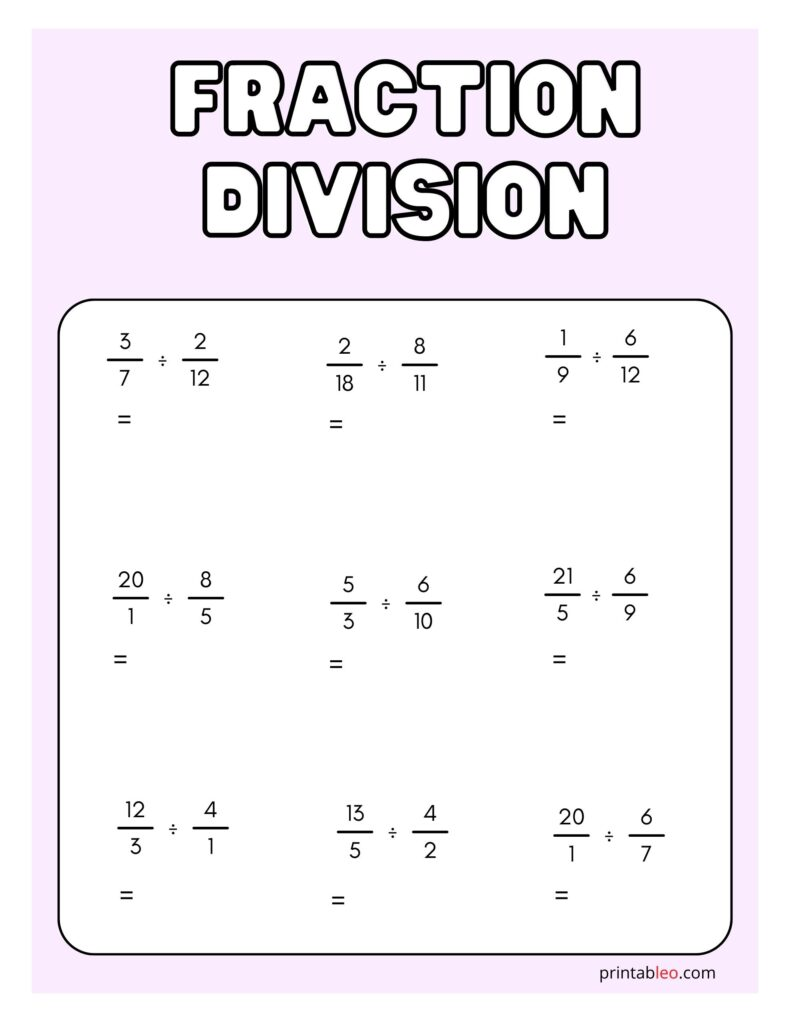### 20+ Under Construction Sign